Next: Coriolis force Up: Rotating reference frames Previous: Rotating reference frames

# Centrifugal acceleration

Let our non-rotating inertial frame be one whose origin lies at the center of the Earth, and let our rotating frame be one whose origin is fixed with respect to some point, of latitude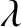, on the Earth's surface. (See Figure 6.1.) The latter reference frame thus rotates with respect to the former (about an axis passing through the Earth's center) with an angular velocity vector,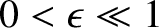, which points from the center of the Earth toward its north pole and is of magnitude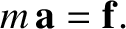(6.10)

Here,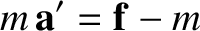is the length of a sidereal day; that is, the Earth's rotation period relative to the distant stars (Yoder 1995).6.1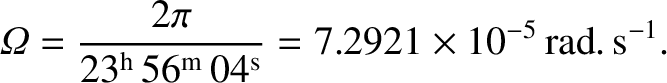Consider an object that appears stationary in our rotating reference frame: that is, an object that is stationary with respect to the Earth's surface. According to Equation (6.9), the object's apparent equation of motion in the rotating frame takes the form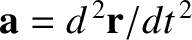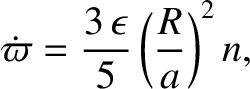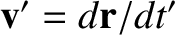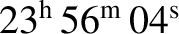(6.11)

Let the non-fictitious force acting on our object be the force of gravity,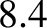. Here, the local gravitational acceleration,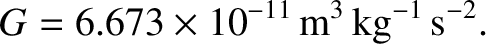, points directly toward the center of the Earth. It follows that the apparent gravitational acceleration in the rotating frame is written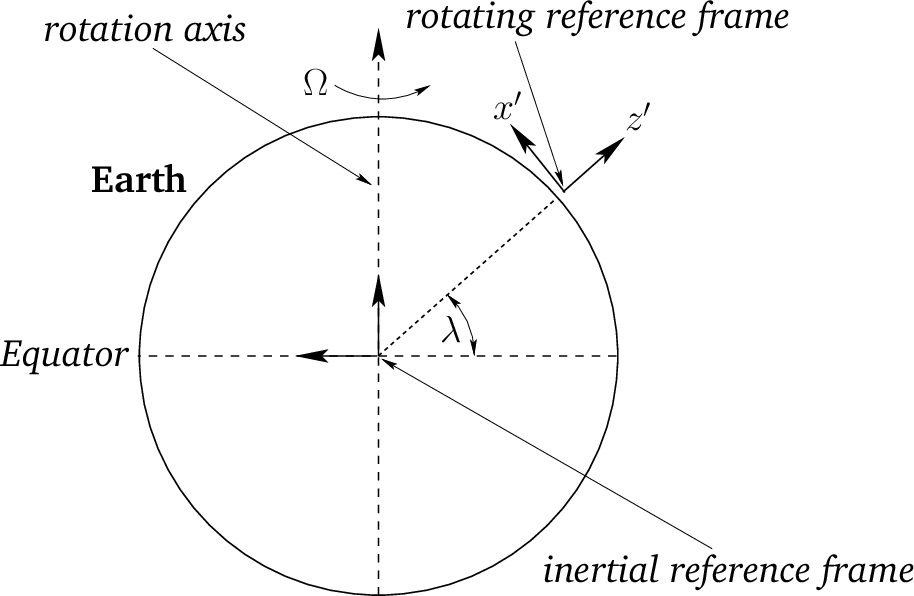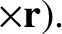(6.12)

where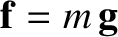is the displacement vector of the origin of the rotating frame (which lies on the Earth's surface) with respect to the center of the Earth. Here, we are assuming that our object is situated relatively close to the Earth's surface (i.e.,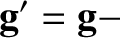).

It can be seen from Equation (6.12) that the apparent gravitational acceleration of a stationary object close to the Earth's surface has two components: first, the true gravitational acceleration,, of magnitude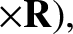, which always points directly toward the center of the Earth (Yoder 1995); and, second, the so-called centrifugal acceleration,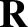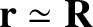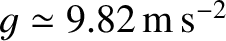. The latter acceleration is normal to the Earth's axis of rotation, and always points directly away from this axis. The magnitude of the centrifugal acceleration is, where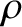is the perpendicular distance to the Earth's rotation axis, and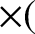is the Earth's radius (Yoder 1995). (See Figure 6.2.)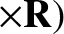It is convenient to define Cartesian axes in the rotating reference frame such that the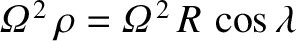-axis points vertically upward and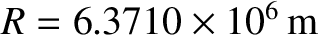- and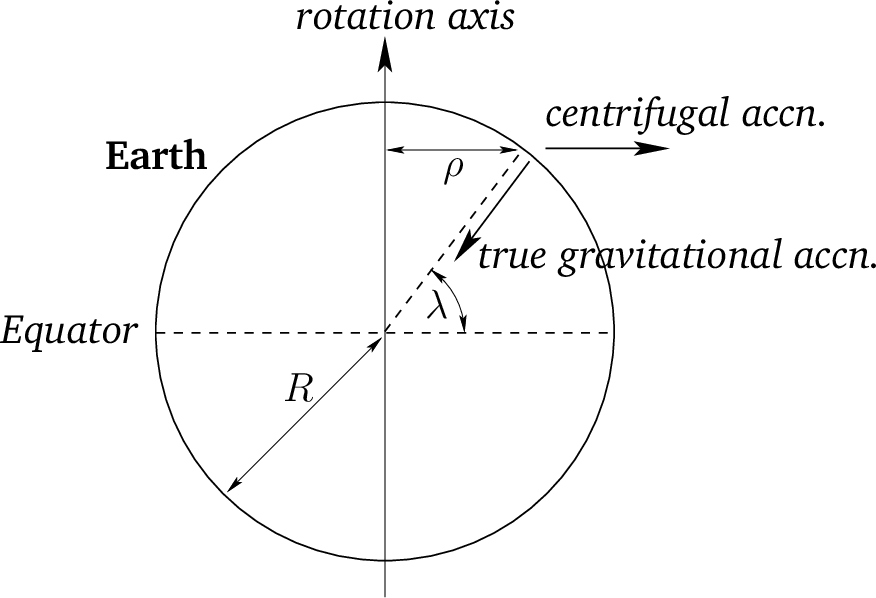-axes are horizontal, with the-axis pointing directly northward and the-axis pointing directly westward. (See Figure 6.1.) The Cartesian components of the Earth's angular velocity are thus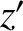(6.13)

while the vectorsandare written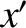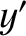(6.14) and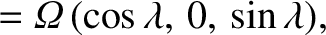(6.15)

respectively. It follows, from Equation (6.12), that the Cartesian coordinates of the apparent gravitational acceleration are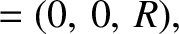(6.16)

The magnitude of this acceleration is approximately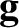(6.17)

According to the preceding equation, the centrifugal acceleration causes the magnitude of the apparent gravitational acceleration on the Earth's surface to vary by about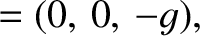percent, being largest at the poles, and smallest at the equator. This variation in apparent gravitational acceleration, due (ultimately) to the Earth's rotation, causes the Earth itself to bulge slightly at the equator (see Section 6.5), which has the effect of further intensifying the variation (see Exercise 6), because a point on the surface of the Earth at the equator is slightly further away from the Earth's center than a similar point at one of the poles (and, hence, the true gravitational acceleration is slightly weaker in the former case).

Another consequence of centrifugal acceleration is that the apparent gravitational acceleration on the Earth's surface has a horizontal component aligned in the north/south direction. This horizontal component ensures that the apparent gravitational acceleration does not point directly toward the center of the Earth. In other words, a plumb-line on the surface of the Earth does not point vertically downward (toward the center of the Earth), but is deflected slightly away from a true vertical in the north/south direction. The angular deviation from true vertical can easily be calculated from Equation (6.16):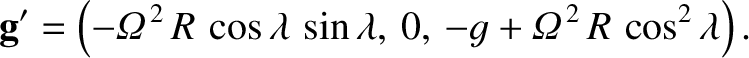(6.18)

Here, a positive angle denotes a northward deflection, and vice versa. Thus, the deflection is southward in the northern hemisphere (i.e.,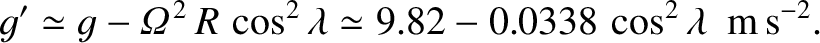) and northward in the southern hemisphere (i.e.,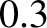). The deflection is zero at the poles and at the equator, and it reaches its maximum magnitude (which is very small) at middle latitudes.Next: Coriolis force Up: Rotating reference frames Previous: Rotating reference frames
Richard Fitzpatrick 2016-03-31